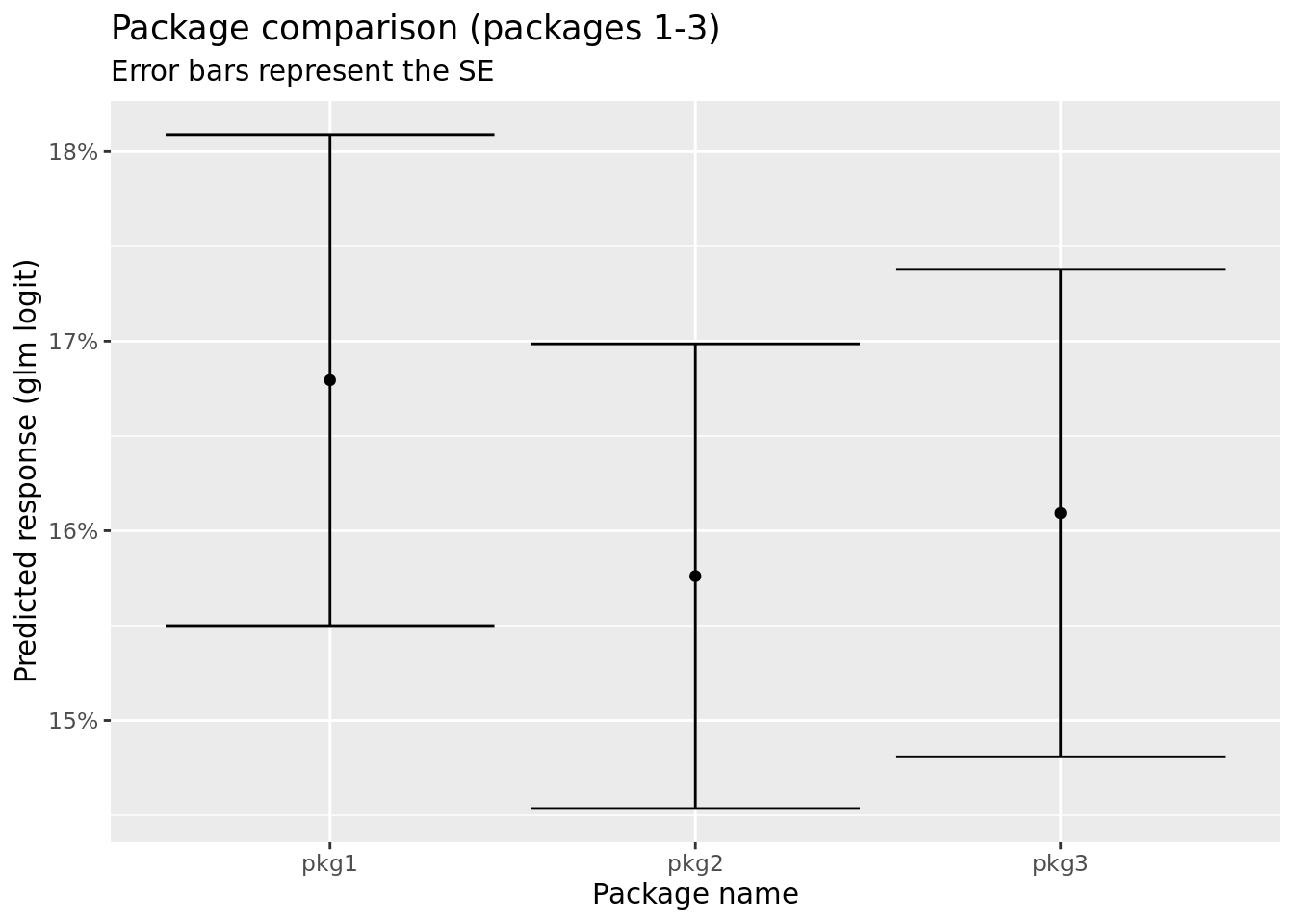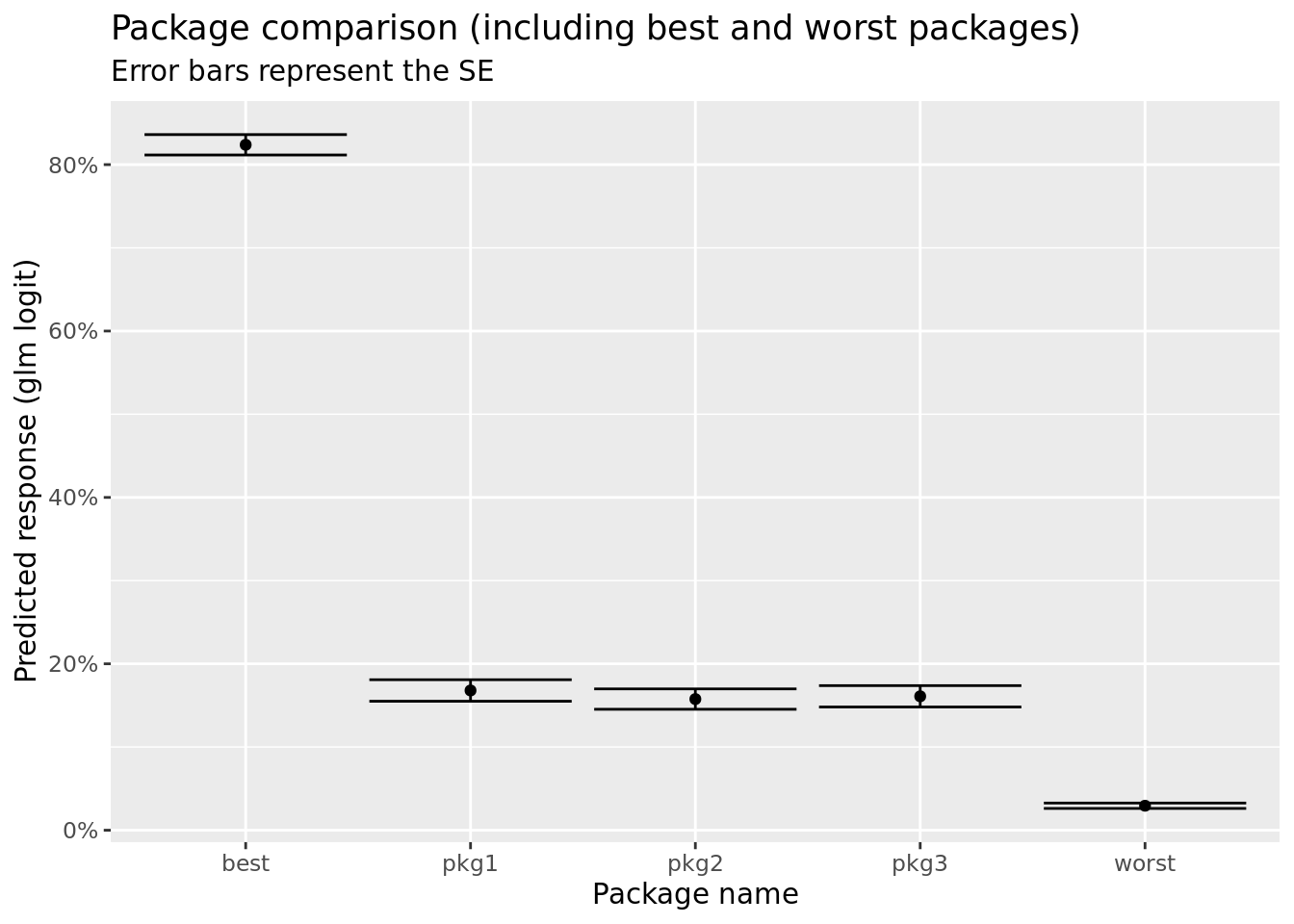# Settling class action lawsuits with conjoint analysis and R (+a conjoint shiny app)

A few days ago I presented at the 9th Israeli class action lawsuit conference. You’re probably asking yourself what would a data scientist do in a room full of lawyers?

Apparently, there is a lot to do… Here’s the story: being in market research, we get a lot of lawyers which are faced with class action lawsuits (either suing or being sued) - and they hire us to conduct research and estimate things like the size of the group for the class action, or the total damages applied on the group.

This time, we did something special. we conducted our own survey, with consumers in the general public in Israel. The goal was to rate various ways of getting compensation (after settling a class action lawsuit).

For that we used conjoint analysis. Conjoint is where you ask the survey participants a set of questions (five in our case). Each question has a number of alternatives (or packages) to choose from, and these are randomized per respondent. In our case we showed three packages, each package is defined by three parameters relating to how a consumer can get compensation in case of a class action being won:

1. Push versus pull - do you have to ask for the compensation or would you get the notification/compensation without asking.
2. The value of the compensation - tested at 4 levels (25, 50, 75, and 100 ILS)
3. The method of delivery - as a complimentary product, a refund at next purchase, bank cheque, or credit card.

The thing about conjoint analysis is that when you diversify enough, you can then run various models to estimate the weight of each parameter, i.e., using logistic regression.

The data is available in the github repo, and the specific data is under the data folder.

``````#library(tidyverse)
skip = 1,
col_names =
c("Response ID", "Set Number", "Card Number",
"compensation_push_pull", "compensation_amount_ILS", "compensation_type",
"score_selection"))``````
``````##
## ── Column specification ────────────────────────────────────────────────────────
## cols(
##   `Response ID` = col_double(),
##   `Set Number` = col_double(),
##   `Card Number` = col_double(),
##   compensation_push_pull = col_character(),
##   compensation_amount_ILS = col_double(),
##   compensation_type = col_character(),
##   score_selection = col_double()
## )``````
``glimpse(class_action_conjoint)``
``````## Rows: 7,020
## Columns: 7
## \$ `Response ID`           <dbl> 10, 10, 10, 10, 10, 10, 10, 10, 10, 10, 10, 10…
## \$ `Set Number`            <dbl> 1, 1, 1, 2, 2, 2, 3, 3, 3, 4, 4, 4, 5, 5, 5, 1…
## \$ `Card Number`           <dbl> 1, 2, 3, 1, 2, 3, 1, 2, 3, 1, 2, 3, 1, 2, 3, 1…
## \$ compensation_push_pull  <chr> "push", "push", "push", "push", "pull", "pull"…
## \$ compensation_amount_ILS <dbl> 75, 50, 25, 100, 25, 100, 75, 100, 50, 25, 75,…
## \$ compensation_type       <chr> "another_product", "credit_cart", "bank_cheque…
## \$ score_selection         <dbl> 0, 0, 100, 0, 100, 0, 0, 0, 100, 0, 100, 0, 10…``````
``class_action_conjoint %>% count(compensation_push_pull)``
``````## # A tibble: 2 x 2
##   compensation_push_pull     n
##   <chr>                  <int>
## 1 pull                    3516
## 2 push                    3504``````
``class_action_conjoint %>% count(compensation_amount_ILS)``
``````## # A tibble: 4 x 2
##   compensation_amount_ILS     n
##                     <dbl> <int>
## 1                      25  1759
## 2                      50  1761
## 3                      75  1752
## 4                     100  1748``````
``class_action_conjoint %>% count(compensation_type)``
``````## # A tibble: 5 x 2
##   compensation_type        n
##   <chr>                <int>
## 1 another_product       1391
## 2 bank_cheque           1410
## 3 coupon                1403
## 4 credit_cart           1415
## 5 refund_next_purchase  1401``````

You can see that the different options are balanced (they should be - they were selected randomly) and that the number of observations is \(7,020\). This is because we had \(n=468\) respondents answering the conjoint question groups, each selecting best one out of three, with five such random sets (\(5*3*468=7020\)).

## Logistic regression

The easiest (and most basic) way to start analyzing the conjoint data is with logistic regression. Note that I’m not endorsing this use of logistic regression in conjoint analysis, because nowadays it has become a standard to compensate for mixed effects (see package `lme4`). However, for the purposes of this post, I’m going to carry on with the simple `glm` which is sufficiently good for our illustration. In any case, my experience is that the models yield similar results in most cases.

``````glm_set <- class_action_conjoint %>%
mutate(score_selection = score_selection/100) %>%
mutate(compensation_push_pull = factor(compensation_push_pull,
levels = c("pull", "push"),
ordered = F),
compensation_type = factor(compensation_type,
levels =
c("another_product",
"refund_next_purchase",
"coupon",
"bank_cheque",
"credit_cart"),
ordered = F)) %>%
select(-`Set Number`, -`Card Number`, -`Response ID`) %>%
mutate(compensation_amount_ILS = factor(compensation_amount_ILS, levels = c(25, 50, 75, 100)))

conjoint_glm_model <- glm(data = glm_set %>%
select(score_selection, compensation_push_pull, compensation_amount_ILS, compensation_type),
formula = score_selection ~ .,
family = binomial())
summary(conjoint_glm_model)``````
``````##
## Call:
## glm(formula = score_selection ~ ., family = binomial(), data = glm_set %>%
##     select(score_selection, compensation_push_pull, compensation_amount_ILS,
##         compensation_type))
##
## Deviance Residuals:
##     Min       1Q   Median       3Q      Max
## -1.8635  -0.8111  -0.5139   0.8796   2.6557
##
## Coefficients:
##                                       Estimate Std. Error z value Pr(>|z|)
## (Intercept)                           -3.49667    0.11341 -30.832   <2e-16 ***
## compensation_push_pullpush             0.74140    0.05807  12.767   <2e-16 ***
## compensation_amount_ILS50              1.01476    0.09385  10.813   <2e-16 ***
## compensation_amount_ILS75              1.73623    0.09224  18.823   <2e-16 ***
## compensation_amount_ILS100             2.40149    0.09281  25.876   <2e-16 ***
## compensation_typerefund_next_purchase  0.08431    0.10399   0.811    0.418
## compensation_typecoupon                1.10396    0.09588  11.514   <2e-16 ***
## compensation_typebank_cheque           1.53888    0.09473  16.245   <2e-16 ***
## compensation_typecredit_cart           1.89640    0.09586  19.782   <2e-16 ***
## ---
## Signif. codes:  0 '***' 0.001 '**' 0.01 '*' 0.05 '.' 0.1 ' ' 1
##
## (Dispersion parameter for binomial family taken to be 1)
##
##     Null deviance: 8936.7  on 7019  degrees of freedom
## Residual deviance: 7295.6  on 7011  degrees of freedom
## AIC: 7313.6
##
## Number of Fisher Scoring iterations: 4``````

Note how most variables (actually all but `compensation_typerefund_next_purchase`) are significant and with a positive estimate (i.e., odds ratio > 1). This means means that when a certain variable increases, the probability of choosing the package increases, i.e.:

• Passively getting the compensation (“Push”) is better than a required act to get the compensation (“pull”) .
• Any sum of money (50, 75, 100) is better than 25, in an increasing odds ratio.
• Most compensation types (credit card payback, bank cheque, coupon) are significantly better than a complimentary product.

Now comes the interesting part: for example, compare the following three packages. Try to guess which one is more attractive:

Parameter Package 1 Package 2 Package 3
Push/Pull Pull Pull Push
Return Credit Refund Coupon
Price 25 75 25

It is not that easy to determine between the three. In this situation there is no single strategy which is superior to the others, we can however plot these three packages with the logistic regression response and standard errors. First let’s put them all in a tibble (I also added the best and worst packages).

``````package_comparison <- tribble(
~package_name, ~compensation_push_pull, ~compensation_amount_ILS, ~compensation_type,
"pkg1", "pull", 25, "credit_cart",
"pkg2", "pull", 75, "refund_next_purchase",
"pkg3", "push", 25, "coupon",
"worst", "pull", 25, "another_product",
"best", "push", 100, "credit_cart"
) %>%
mutate(compensation_amount_ILS = factor(compensation_amount_ILS)) # need to convert to factor - which is how it is modeled in the glm.

predicted_responses <- predict(conjoint_glm_model, newdata = package_comparison, type = "response", se.fit = T)

# lets join these together

package_responses <- package_comparison %>%
mutate(fit = predicted_responses\$fit,
se.fit = predicted_responses\$se.fit)

package_responses``````
``````## # A tibble: 5 x 6
##   package_name compensation_pu… compensation_am… compensation_ty…    fit  se.fit
##   <chr>        <chr>            <fct>            <chr>             <dbl>   <dbl>
## 1 pkg1         pull             25               credit_cart      0.168  0.0129
## 2 pkg2         pull             75               refund_next_pur… 0.158  0.0122
## 3 pkg3         push             25               coupon           0.161  0.0129
## 4 worst        pull             25               another_product  0.0294 0.00324
## 5 best         push             100              credit_cart      0.824  0.0123``````
``````# P.S. - excuse the "credit_cart" typo (I build the model that way, only then noticed...)

ggplot(package_responses %>% slice(1:3) , aes(x = package_name, y = fit)) +
geom_point() +
geom_errorbar(aes(ymin = fit - se.fit, ymax = fit + se.fit)) +
ggtitle("Package comparison (packages 1-3)", subtitle = "Error bars represent the SE") +
ylab("Predicted response (glm logit)") +
xlab("Package name") +
scale_y_continuous(labels = scales::percent_format(accuracy = 1))````````````ggplot(package_responses, aes(x = package_name, y = fit)) +
geom_point() +
geom_errorbar(aes(ymin = fit - se.fit, ymax = fit + se.fit)) +
ggtitle("Package comparison (including best and worst packages)", subtitle = "Error bars represent the SE") +
ylab("Predicted response (glm logit)") +
xlab("Package name") +
scale_y_continuous(labels = scales::percent_format(accuracy = 1))``````We see that the three packages (pkg1, pkg2, and pkg3) are relatively similar, within one standard error from one another. When compared to the worst package they are roughly \(\sim8\) times better (via odds ratio), but the best package is \(\sim5\) times better than packages 1-3.

One can use these concepts to illustrate the benefits of each parameter on the different packages, and let the user experience how different features make the packages more or less “attractive”.

As an experiment, I prepared a nice little shiny app which lets the user experiment with the different features: build two packages and then compare them. You can checkout the code at the github repo, or check out the live app here.

## Conclusions

Surveys are a popular tool used in class actions (at least in Israel). They can be used to estimate the tradeoffs between various types of compensation or settlement, for example with the use of conjoint analysis.

With a glm model one can tell the differences of various packages, and the odds ratio is a way to illustrate to decision makers a comparison of various options (and how much “more attractive” is one package over another).

A shiny app can be a nice way to illustrate the results of a conjoint analysis, and to let the user experiment with how different features make a specific option better or worse than another option.